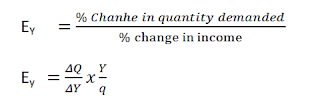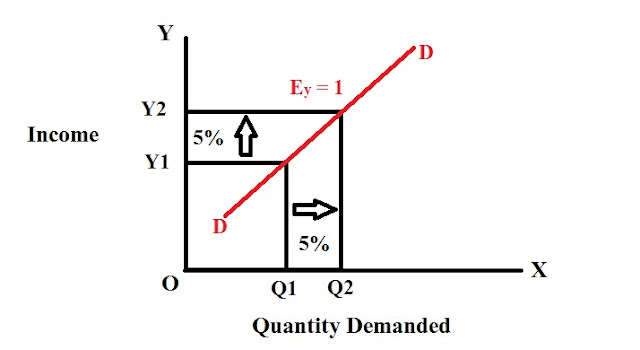Income elasticity of demand Income elasticity of demand

# Income elasticity of demand

## Income elasticity of demand

The income of a consumer is the most influencing factor of demand for a good. Demand for goods responds to the change in the income of the buyer. The measure of the responsiveness of a quantity demanded of a product to the change in the income of the buyer, other things being constant is known as income elasticity of demand. The value of it may be positive, negative, or zero depending on the nature of the commodity. Income elasticity is usually symbolized by EY. It can be expressed as:Where
∆Q=change in quantity demanded
∆Y=change in income
Y=Initial income
Q=initial quantity demanded

### Degrees/Types of income elasticity of demand

There are three types of income elasticity of demand. They are as follows:-

1. Zero income elasticity of demand(EY=0):
If the quantity demanded for a commodity is totally irresponsive to the change in income of the consumer, then the demand is known as zero income elasticity of demand. There is no relationship between the change in income and demand.

In the above figure, income and quantity are shown along the y-axis and x-axis respectively. When income increases from I to I1 or decreases from I to IO, the quantity demanded remains constant I.e. OQ. DD is a demand curve that shows zero elasticity I.e. EY= O.

2. Positive income elasticity of demand (EY>0): When quantity demanded increases with the increase in consumer’s income and decreases with a decrease in consumer’s income, then it is the case of Positive income elasticity of demand. Here the value of elasticity of demand remains greater than 0.

In the above figure income and quantity are shown along the y-axis and x-axis respectively. When income increases from Y to Y1, the quantity demanded increases from Q1 to Q2. DD is the demand curve that is positively elastic in nature.I .e EY > 0

Positive income elasticity of demand can be further divided into the following three types:

a. Income elasticity greater than unity ( EY > 1):
When the percentage increase in quantity demanded is greater than the percentage increase in income and vice versa, then the income elasticity is said to be greater than unity. For example, if income increases by 5%, quantity demanded increases by 10%. It can be graphically shown as follows:In the above graph, we can observe that when income increases from OY1 to OY2 by 5%, quantity demanded increases from OQ1 to OQ2 by 10%. DD is the demand curve that shows income elasticity greater than unity.

b. Income elasticity less than unity ( EY < 1):
When the percentage increase in quantity demanded is less than the percentage increase in income and vice versa, then the income elasticity is said to be less than unity. For example, if income increases by 10%, quantity demanded increases only by 5%. It can be graphically shown as follows:In the above graph, we can observe that when income increases from OY1 to OY2 by 10%, quantity demanded increases from OQ1 to OQ2 by 5%. DD is the demand curve that shows income elasticity less than unity.

b. Income elasticity equal to unity ( EY = 1):
When the percentage increase in quantity demanded is equal to the percentage increase in income, then the income elasticity is said to be equal to unity. For example, if income increases by 5%, quantity demanded also increases by 5%. It can be graphically shown as follows:In the above graph, we can observe that when income increases from OY1 to OY2 by 5%, quantity demanded also increases from OQ1 to OQ2 by 5%. DD is the demand curve that shows income elasticity equal to unity.

3. Negative income elasticity of demand (EY<0)
When demand for any commodity decreases with the increase in consumer’s income and vice versa. Then it is the case of negative income elasticity of demand. Here the value of elasticity of demand remains less than 0.

In the above figure, income and quantity are shown along the y-axis and x-axis respectively. When income decreases from I to I0, the quantity demanded increases from Q to Q1. DD is the demand curve that is negatively elastic in nature. I.e. EY < 0

Income elasticity of demand can also be presented in the following combined diagram:

# Second Hand Dell Laptop for Sale in Nepal

Specifications:

Brand: Dell

Display: 15.6 inches

Processor: Ryzen 7 (i7) 11th gen

RAM: 16GB DDR4

Storage: 512GB NVME SSD

Operating System: Windows 11, 64 Bit

Used for: 1 Year

Reason for Sale: Buying a Macbook.

Price: Rs. 91,000 only